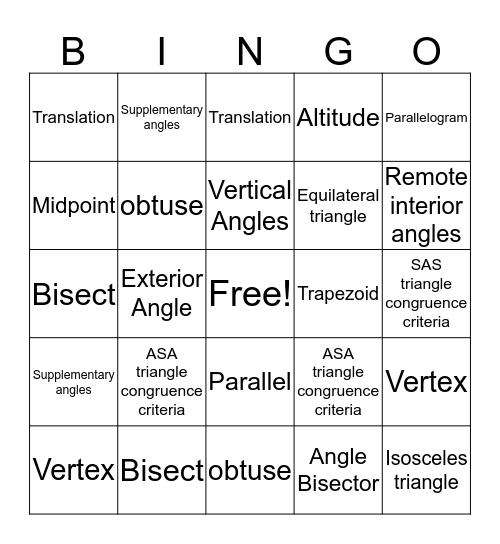# Module 5 Vocabulary BingoThis bingo card has a free space and 54 words: Linear Pairs, Supplementary angles, Rhombus, Perpendicular Bisector of a side, Parallel, Angle Bisector, Altitude, Median, Midpoint, Rotation, Translation, Reflection, Bisect, Vertex, Acute, obtuse, Isosceles triangle, Equilateral triangle, SSS triangle congruence criteria, SAS triangle congruence criteria, ASA triangle congruence criteria, Congruent, Trapezoid, Parallelogram, Linear Pairs, Supplementary angles, Rhombus, Perpendicular Bisector of a side, Parallel, Angle Bisector, Altitude, Median, Midpoint, Rotation, Translation, Reflection, Bisect, Vertex, Acute, obtuse, Isosceles triangle, Equilateral triangle, SSS triangle congruence criteria, SAS triangle congruence criteria, ASA triangle congruence criteria, Congruent, Trapezoid, Parallelogram, Vertical Angles, Exterior Angle, Remote interior angles, Transversal line, Corresponding angles and Alternate interior angles.

⚠ This card has duplicate items: Linear Pairs (2), Supplementary angles (2), Rhombus (2), Perpendicular Bisector of a side (2), Parallel (2), Angle Bisector (2), Altitude (2), Median (2), Midpoint (2), Rotation (2), Translation (2), Reflection (2), Bisect (2), Vertex (2), Acute (2), obtuse (2), Isosceles triangle (2), Equilateral triangle (2), SSS triangle congruence criteria (2), SAS triangle congruence criteria (2), ASA triangle congruence criteria (2), Congruent (2), Trapezoid (2), Parallelogram (2)

## Play Online